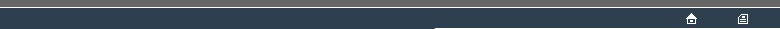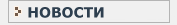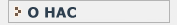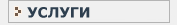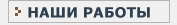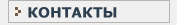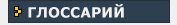СТАТИСТИЧЕСКИЙ В данном разделе Вы найдете все интересующие Вас термины и определения, связанные с статистикой и статистическими исследованиями.КЛИНИЧЕСКИЕ ИССЛЕДОВАНИЯ Необходимый перечень терминов и определений, используемых при проведении клинических испытанийN NOMINAL ALPHA CRITERION LEVEL A publicly agreed value for TYPE-1 ERROR, such that the outcome of a statistical test is classified in terms of whether the obtained value of ALPHA is extreme as compared with this criterion level. The fine detail of the comparison involves the TAIL DEFINITION POLICY. The outcome is classified as showing STATISTICAL SIGNIFICANCE ('significant') if the outcome has low ALPHA as compared with the NOMINAL ALPHA CRITERION LEVEL, otherwise not ('non-significant'). The commonest conventional values for the NOMINAL ALPHA CRITERION LEVEL are 0.05 and 0.01 . NOMINAL SCALE This is a type of MEASUREMENT SCALE with a limited number of possible outcomes which cannot be placed in any order representing the intrinsic properties of the measurements. Examples : Female versus Male; the collection of languages in which an international treaty is published. NON-PARAMETRIC TEST A number of statistical tests were devised, mostly over the period 1930-1960, with the specific objective of by-passing assumptions about sampling from populations with data supposedly conforming to theoretically modelled statistical distributions wuch as the NORMAL DISTRIBUTION. Several of these tests were explictly concerned with ORDINAL-SCALE data for which modelling based upon continuous functions is clearly inappropriate. These tests are implicitly RE-RANDOMISATION TESTS. Also see : BINOMIAL TEST, MANN-WHITNEY TEST, WILCOXON TEST(1 and 2). NORMAL DISTRIBUTION [] The NORMAL DISTRIBUTION is a theoretical distribution applicable for continuous INTERVAL-SCALE data. It is related mathematically to the BINOMIAL and CHI-SQUARE(2) distributions and to several named sampling distributions (including Student's t, Fisher's F, Pearson's r); these sampling distributions are the characteristic tools of parametric statisical infernece to which RE-RANDOMISATION STATISTICS are an alternative. NULL HYPOTHESIS In order to test whether a supposed interesting pattern exists in a set of data, it is usual to propose a NULL HYPOTHESIS that the pattern does not exist. It is the unexpectedness of the degree of departure of the observed data, relative to the pattern expected under the NULL HYPOTHESIS, which is examined by the measure ALPHA. Reference to a NULL HYPOTHESIS is common between RE-RANDOMISATION STATISTICS and parametric statistics. Also see : BETA.N
NOMINAL ALPHA CRITERION LEVEL
NOMINAL SCALE
NON-PARAMETRIC TEST
NORMAL DISTRIBUTION
NULL HYPOTHESISНовости   О нас   Услуги   Наши работы   Статьи   Контакты   Глоссарий
Статистическая помощь! © 2005 - 2022 • Защита авторских прав